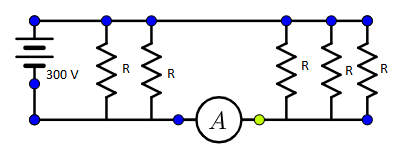# Five identical resistors each of resistance $R = 1500\,\Omega$ are connected to a $300\,V$ battery as shown in the circuit. The reading of the ideal ammeter $A$ is(A) $\dfrac{1}{5}\,A$ (B) $\dfrac{3}{5}\,A$ (C) $\dfrac{2}{5}\,A$ (D) $\dfrac{4}{5}\,A$Verified
176.1k+ views
Hint : The current value shown in the ammeter is determined by using the ohm’s law formula. The resistance value which is substituted in the ohm's law is the total resistance. The total resistance is determined by using the resistor in parallel formula.

Useful formula:
The ohm's law states that,
$V = IR$
Where, $V$ is the voltage, $I$ is the current and $R$ is the resistance.
The resistance in parallel formula is given by,
$\dfrac{1}{{{R_T}}} = \dfrac{1}{{{R_1}}} + \dfrac{1}{{{R_2}}} + \dfrac{1}{{{R_3}}} + \dfrac{1}{{{R_4}}} + \dfrac{1}{{{R_5}}}$
Where, ${R_T}$ is the total resistance and ${R_1}$ , ${R_2}$ etc. are the resistance connected in parallel.

Given that,
The resistance all are equal, so ${R_1} = {R_2} = {R_3} = {R_4} = {R_5} = R = 1500\,\Omega$
The voltage in the circuit is, $V = 300\,V$
Now,
The resistance in parallel formula is given by,
$\dfrac{1}{{{R_T}}} = \dfrac{1}{{{R_1}}} + \dfrac{1}{{{R_2}}} + \dfrac{1}{{{R_3}}} + \dfrac{1}{{{R_4}}} + \dfrac{1}{{{R_5}}}\,..................\left( 1 \right)$
By substituting the value of the equal resistance of the five resistance are connected in parallel in the above equation (1), then the above equation (1) is written as,
$\dfrac{1}{{{R_T}}} = \dfrac{1}{R} + \dfrac{1}{R} + \dfrac{1}{R} + \dfrac{1}{R} + \dfrac{1}{R}$
By adding the terms in the above equation, then the above equation is written as,
$\dfrac{1}{{{R_T}}} = \dfrac{5}{R}$
By taking the reciprocal on both sides, then the above equation is written as,
${R_T} = \dfrac{R}{5}$
Now,
The ohm's law states that,
$V = IR\,................\left( 2 \right)$
In this equation the $R$ is the total resistance, so by substituting the voltage and total resistance in the above equation (2), then the equation (2) is written as,
$300 = I \times \dfrac{R}{5}$
By rearranging the terms, then
$\dfrac{{300 \times 5}}{R} = I$
By substituting the resistance values in the above equation, then
$I = \dfrac{{300 \times 5}}{{1500}}$
On multiplying the terms, then
$I = \dfrac{{1500}}{{1500}}$
By cancelling the terms, then
$I = 1\,A$
The answer is not matched with the option given in the question.

Note
The five resistance are of equal resistance, so the value is not substituted in the resistor in parallel formula. If the five resistors are of different resistance, the value of the five different resistors must be substituted in the resistor in parallel formula. At the condition of the five-resistor having different resistance, this type of calculation is not possible.